Today I am writing a little blog about SQL joins

First we have to create two tables and insert some very simple data for testing.

```CREATE TABLE [dbo].[TableLeft]([Key] [varchar](50) NULL,
[LeftValue] [varchar](50) NULL)

CREATE TABLE [dbo].[TableRight]([Key] [varchar](50) NULL,
[RightValue] [varchar](50) NULL)

INSERT [dbo].[TableLeft] ([Key], [LeftValue]) VALUES (N'3', N'LeftValue_1')
INSERT [dbo].[TableLeft] ([Key], [LeftValue]) VALUES (N'1', N'LeftValue_2')
INSERT [dbo].[TableRight] ([Key], [RightValue]) VALUES (N'1', N'RightValue_1')
INSERT [dbo].[TableRight] ([Key], [RightValue]) VALUES (N'2', N'RightValue_2')
```

Cartesian product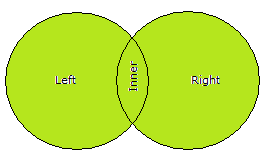A Cartesian join is when you join every row of one table to every row of another table.
You can also get one by joining every row of a table to every row of itself.

```SELECT l.*,
r.*
FROM [dbo].[TableLeft] l,
[dbo].[TableRight] r
```

The result looks like this: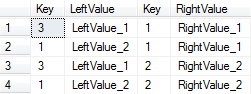INNER JOIN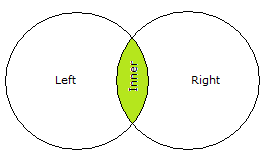An INNER JOIN is the most common join operation used in applications and can be regarded as the default join-type.
Inner join creates a new result table by combining column values of two tables (A and B) based upon the join-predicate.
The query compares each row of A with each row of B to find all pairs of rows which satisfy the join-predicate.
When the join-predicate is satisfied, column values for each matched pair of rows of A and B are combined into a result row.
The result of the join can be defined as the outcome of first taking the Cartesian product (or cross-join)
of all records in the tables (combining every record in table A with every record in table B)
-then return all records which satisfy the join predicate.
Actual SQL implementations normally use other approaches like a hash join or a sort-merge join where possible,
since computing the Cartesian product is very inefficient.

```SELECT l.*,
r.*
FROM [dbo].[TableLeft] l,
[dbo].[TableRight] r
WHERE l.[Key] = r.[Key]

SELECT l.*,
r.*
FROM [dbo].[TableLeft] l
JOIN [dbo].[TableRight] r
ON l.[Key] = r.[Key]

SELECT l.*,
r.*
FROM [dbo].[TableLeft] l
INNER JOIN [dbo].[TableRight] r
ON l.[Key] = r.[Key]

SELECT l.*
FROM [dbo].[TableLeft] l
WHERE l.[Key] = (SELECT [Key]
FROM [dbo].[TableRight]
WHERE [Key] = l.[Key])
```

The result looks like this: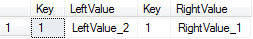LEFT OUTER JOIN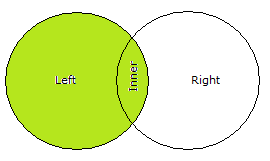The result of a left outer join (or simply LEFT JOIN) for table A and B always contains all records of the “left” table (A),
even if the join-condition does not find any matching record in the “right” table (B).
This means that if the ON clause matches 0 (zero) records in B, the join will still return a row in the result—but with NULL
in each column from B. This means that a LEFT OUTER JOIN returns all the values from the left table,
plus matched values from the right table (or NULL in case of no matching join predicate).
If the right table returns one row and the left table returns more than one matching row for it,
the values in the right table will be repeated for each distinct row on the left table.

For example, this allows us to find an employee’s department, but still shows the employee(s) even when they have not been assigned to a
department (contrary to the inner-join example above, where unassigned employees are excluded from the result).

```SELECT l.*,
r.*
FROM [dbo].[TableLeft] l
LEFT OUTER JOIN [dbo].[TableRight] r
ON l.[Key] = r.[Key]
```

The result looks like this: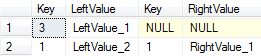Following a wrong LEFT OUTER JOIN, the result of this is a INNER JOIN

```SELECT l.*,
r.*
FROM [dbo].[TableLeft] l
LEFT OUTER JOIN [dbo].[TableRight] r
ON l.[Key] = r.[Key]
WHERE Right(r.[RightValue], 1) = '1'
```

The result looks like this: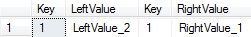Following how the correct LEFT OUTER JOIN would look like.
The key is to add an “OR … is null” to each WHERE clause against the right table.

```SELECT l.*,
r.*
FROM [dbo].[TableLeft] l
LEFT OUTER JOIN [dbo].[TableRight] r
ON l.[Key] = r.[Key]
WHERE (Right(r.[RightValue], 1) = '1'
OR Right(r.[RightValue], 1) is null)
```

The result looks like this: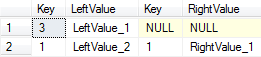RIGHT OUTER JOIN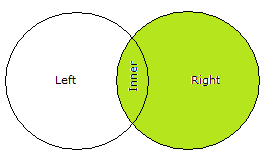A RIGHT OUTER JOIN (or RIGHT JOIN) closely resembles a left outer join, except with the treatment of the tables reversed.
Every row from the “right” table (B) will appear in the joined table at least once.
If no matching row from the “left” table (A) exists, NULL will appear in columns from A for those records that have no match in B.
A right outer join returns all the values from the right table and matched values from the left table
(NULL in case of no matching join predicate).

For example, this allows us to find each employee and his or her department, but still show departments that have no employees.

```SELECT l.*,
r.*
FROM [dbo].[TableLeft] l
RIGHT OUTER JOIN [dbo].[TableRight] r
ON l.[Key] = r.[Key]
```

The result looks like this: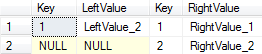Following a wrong RIGHT OUTER JOIN, the result of this is a INNER JOIN

```SELECT l.*,
r.*
FROM [dbo].[TableLeft] l
RIGHT OUTER JOIN [dbo].[TableRight] r
ON l.[Key] = r.[Key]
WHERE Right(l.[LeftValue], 1) = '2'
```

The result looks like this: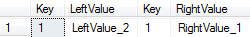Following how the correct RIGHT OUTER JOIN would look like.
The key is to add an “OR … is null” to each WHERE clause against the left table.

```SELECT l.*,
r.*
FROM [dbo].[TableLeft] l
RIGHT OUTER JOIN [dbo].[TableRight] r
ON l.[Key] = r.[Key]
WHERE (Right(l.[LeftValue], 1) = '2'
OR Right(l.[LeftValue], 1) is null)
```

The result looks like this: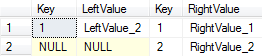FULL OUTER JOINConceptually, a FULL OUTER JOIN combines the effect of applying both left and right outer joins. Where records in the FULL OUTER JOINed tables
do not match, the result set will have NULL values for every column of the table that lacks a matching row. For those records that do match, a
single row will be produced in the result set (containing fields populated from both tables).
For example, this allows us to see each employee who is in a department and each department that has an employee, but also see each employee who
is not part of a department and each department which doesn’t have an employee

```SELECT l.*,
r.*
FROM [dbo].[TableLeft] l
FULL OUTER JOIN [dbo].[TableRight] r
ON l.[Key] = r.[Key]
```

The result looks like this: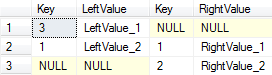Following a wrong FULL OUTER JOIN, the result of this is a INNER JOIN

```SELECT l.*,
r.*
FROM [dbo].[TableLeft] l
FULL OUTER JOIN [dbo].[TableRight] r
ON l.[Key] = r.[Key]
WHERE Right(l.[LeftValue], 1)  = '2'
AND   Right(r.[RightValue], 1) = '1'
```

The result looks like this: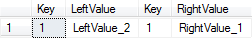Following how the correct FULL OUTER JOIN would look like.
The key is to add an “OR … is null” to each WHERE clause against the left and right table.

```SELECT l.*,
r.*
FROM [dbo].[TableLeft] l
FULL OUTER JOIN [dbo].[TableRight] r
ON l.[Key] = r.[Key]
WHERE (Right(l.[LeftValue], 1) = '2'
OR Right(l.[LeftValue], 1) is null)
OR    (Right(r.[RightValue], 1) = '1'
OR Right(r.[RightValue], 1) is null)
```

The result looks like this: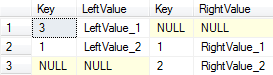CROSS JOIN

SQL CROSS JOIN will return all records where each row from the first table is combined with each row from the second table.
Which also mean CROSS JOIN returns the Cartesian product of the sets of rows from the joined tables.
A CROSS JOIN can be specified in two ways: using the JOIN syntax or by listing the tables in the FROM clause separated by
commas without using a WHERE clause to supply join criteria.

```SELECT *
FROM [dbo].[TableLeft],
[dbo].[TableRight]

SELECT *
FROM [dbo].[TableLeft]
CROSS JOIN [dbo].[TableRight]
```

Both results are the same: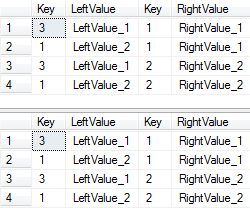Cleanup tables

```DROP TABLE [dbo].[TableLeft]
DROP TABLE [dbo].[TableRight]
```